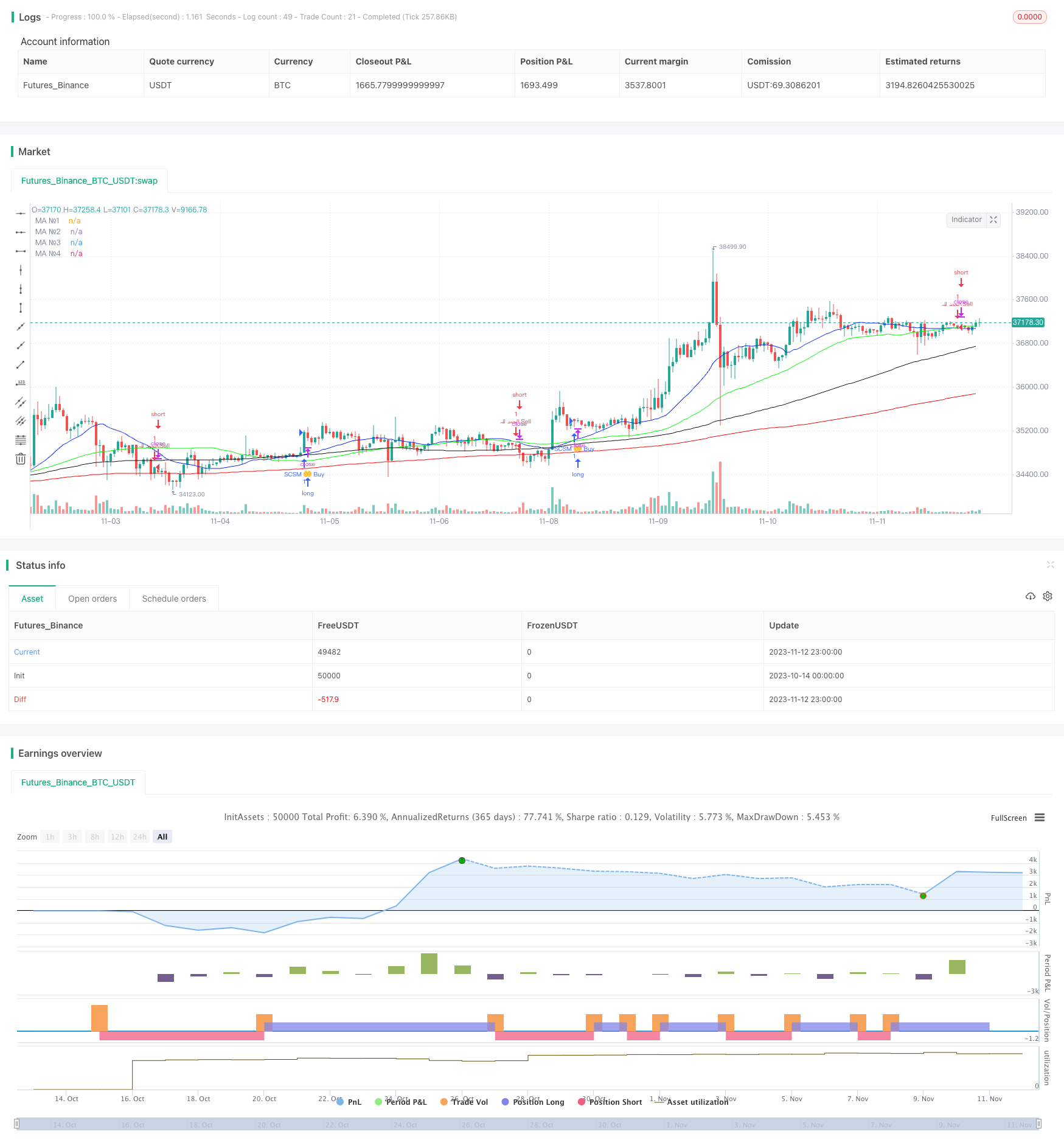# Simple Moving Average Crossover Strategy

Author: ChaoZhang, Date: 2023-11-14 16:17:16
Tags:## Overview

This strategy generates buy and sell signals based on golden cross and death cross of multiple simple moving averages (SMAs) with different time periods to determine the trend direction. It uses 4 SMAs - 20-day, 50-day, 100-day and 200-day SMA. When the shorter-term SMA crosses above the longer-term SMA, it is considered a golden cross and a buy signal is triggered. When the shorter-term SMA crosses below the longer-term SMA, it is considered a death cross and a sell signal is triggered.

## Strategy Logic

The core logic of this strategy is based on the following points:

1. Calculate multiple SMAs with different time periods including 20-day, 50-day, 100-day and 200-day SMA.

2. Check the crossover situations between the shorter-term SMA (20-day) and longer-term SMAs (50-day, 100-day, 200-day).

3. When the 20-day SMA crosses above the 50-day SMA, it is considered a golden cross and a buy signal is triggered. When the 20-day SMA crosses below the 50-day SMA, it is considered a death cross and a sell signal is triggered.

4. The larger trend is determined by the longer time period SMAs staying above the shorter time period SMAs, i.e. 50-day SMA > 20-day SMA.

5. The priority for entry signals is: 20-day SMA vs 50-day SMA > 20-day SMA vs 100-day SMA > 20-day SMA vs 200-day SMA.

6. Exit signal is generated when the 20-day SMA crosses back below the 50-day SMA.

The strategy mainly relies on SMA crossovers to determine the trend direction. Golden crosses in bull markets and death crosses in bear markets can signal potential trend start. In addition, longer term SMAs staying above shorter term SMAs serve as confirmation of the larger trend.

The main advantages of this strategy include:

1. The logic is simple and easy to understand and implement.

2. SMAs are better than EMAs in filtering market noise and identifying the trend.

3. Using multiple time period SMAs improves signal reliability.

4. The priority setting for entry signals avoids premature entry.

5. Customizable SMA periods and colors allow strategy optimization.

6. Applicable to multiple timeframes for different trading styles.

7. SMA crossover system is very effective in determining the major trend direction.

## Risks of the Strategy

Some risks associated with this strategy:

1. Too many false signals may occur during ranging markets with frequent SMA crosses.

2. Fixed SMA periods cannot adapt to market changes, parameters should be optimized based on trend and volatility.

3. SMA crosses alone cannot determine precise entry, other indicators like MACD should be incorporated.

4. SMAs have lagging nature, entry timing needs optimization or limit orders should be used.

5. Strict stop loss implementation is crucial for capital preservation.

6. Trading costs impact on profitability should be considered.

## Enhancement of the Strategy

Some ways to optimize this strategy:

1. Optimize SMA periods dynamically based on market conditions and ATR.

2. Add other indicators like MACD, RSI for entry timing.

3. Add trend filter like ADX to avoid false signals during consolidation.

4. Optimize stop loss methods like ATR stop or trailing stop.

5. Manage position sizing dynamically based on account size.

6. Test optimal parameters across different asset classes.

7. Incorporate multiple timeframes to ensure consistency with higher timeframe trend.

## Conclusion

In summary, this simple SMA crossover system is reliable in determining trend direction and suitable for most traders. However, it has some lagging issues and can generate false signals. We should look to enhance entry timing, stop loss, position sizing etc. to make it robust across changing market environments. The combination of multiple technical indicators and trend evaluation is key to building a solid trend following strategy.

```/*backtest
start: 2023-10-14 00:00:00
end: 2023-11-13 00:00:00
period: 1h
basePeriod: 15m
exchanges: [{"eid":"Futures_Binance","currency":"BTC_USDT"}]
*/

// This source code is subject to the terms of the Mozilla Public License 2.0 at https://mozilla.org/MPL/2.0/
//@version=5
strategy("SMA crossover buy/sell [SCSM_Algo]", overlay=true, margin_long=3000, margin_short=3000)

BuyCond = ta.crossover(ta.sma(close, 20), ta.sma(close, 50)) and ta.sma(close, 20) > ta.sma(close, 50) and  ta.sma(close, 50) > ta.sma(close, 100) and  ta.sma(close, 100) > ta.sma(close, 200) or (ta.crossover(ta.sma(close, 20), ta.sma(close, 100)) and ta.sma(close, 20) > ta.sma(close, 50))

SellCond = ta.crossunder(ta.sma(close, 20), ta.sma(close, 50))
if (SellCond)
strategy.entry("الحمد للہ،Sell", strategy.short)

ma(source, length, type) =>
type == "SMA" ? ta.sma(source, length) :
type == "EMA" ? ta.ema(source, length) :
type == "SMMA (RMA)" ? ta.rma(source, length) :
type == "WMA" ? ta.wma(source, length) :
type == "VWMA" ? ta.vwma(source, length) :
na

show_ma1   = input(true   , "MA №1", inline="MA #1")
ma1_type   = input.string("SMA"  , ""     , inline="MA #1", options=["SMA", "EMA", "SMMA (RMA)", "WMA", "VWMA"])
ma1_source = input(close  , ""     , inline="MA #1")
ma1_length = input.int(20     , ""     , inline="MA #1", minval=1)
ma1_color  = input(#0929f6, ""     , inline="MA #1")
ma1 = ma(ma1_source, ma1_length, ma1_type)
plot(show_ma1 ? ma1 : na, color = ma1_color, title="MA №1")

show_ma2   = input(true   , "MA №2", inline="MA #2")
ma2_type   = input.string("SMA"  , ""     , inline="MA #2", options=["SMA", "EMA", "SMMA (RMA)", "WMA", "VWMA"])
ma2_source = input(close  , ""     , inline="MA #2")
ma2_length = input.int(50     , ""     , inline="MA #2", minval=1)
ma2_color  = input(#00fb04, ""     , inline="MA #2")
ma2 = ma(ma2_source, ma2_length, ma2_type)
plot(show_ma2 ? ma2 : na, color = ma2_color, title="MA №2")

show_ma3   = input(true   , "MA №3", inline="MA #3")
ma3_type   = input.string("SMA"  , ""     , inline="MA #3", options=["SMA", "EMA", "SMMA (RMA)", "WMA", "VWMA"])
ma3_source = input(close  , ""     , inline="MA #3")
ma3_length = input.int(100    , ""     , inline="MA #3", minval=1)
ma3_color  = input(#131313, ""     , inline="MA #3")
ma3 = ma(ma3_source, ma3_length, ma3_type)
plot(show_ma3 ? ma3 : na, color = ma3_color, title="MA №3")

show_ma4   = input(true   , "MA №4", inline="MA #4")
ma4_type   = input.string("SMA"  , ""     , inline="MA #4", options=["SMA", "EMA", "SMMA (RMA)", "WMA", "VWMA"])
ma4_source = input(close  , ""     , inline="MA #4")
ma4_length = input.int(200    , ""     , inline="MA #4", minval=1)
ma4_color  = input(#f60c0c, ""     , inline="MA #4")
ma4 = ma(ma4_source, ma4_length, ma4_type)
plot(show_ma4 ? ma4 : na, color = ma4_color, title="MA №4")
```

More